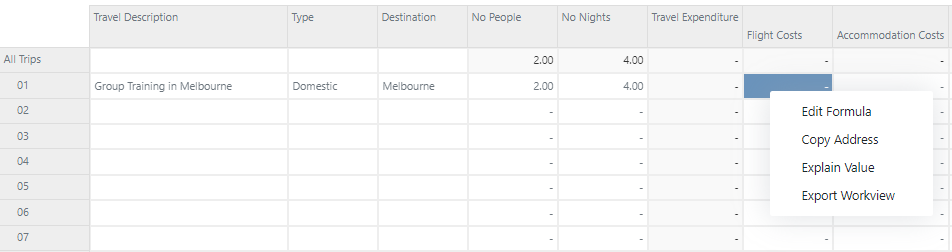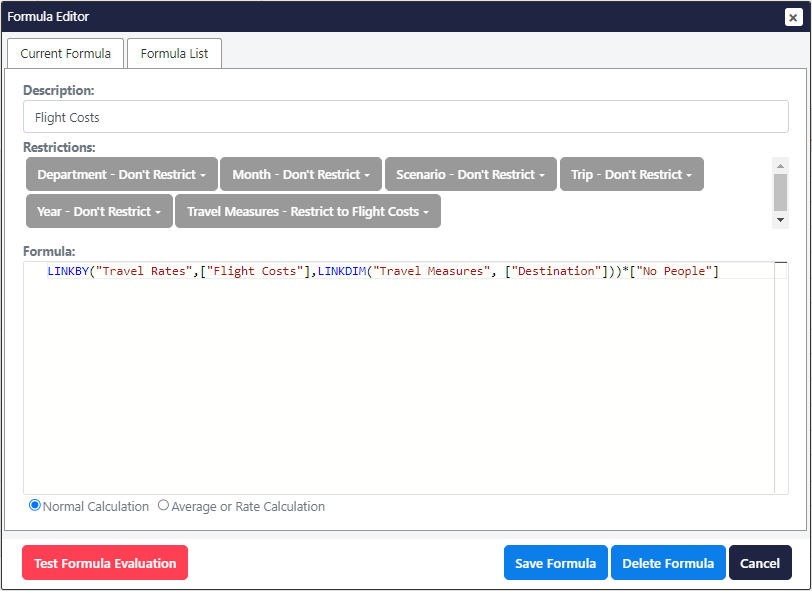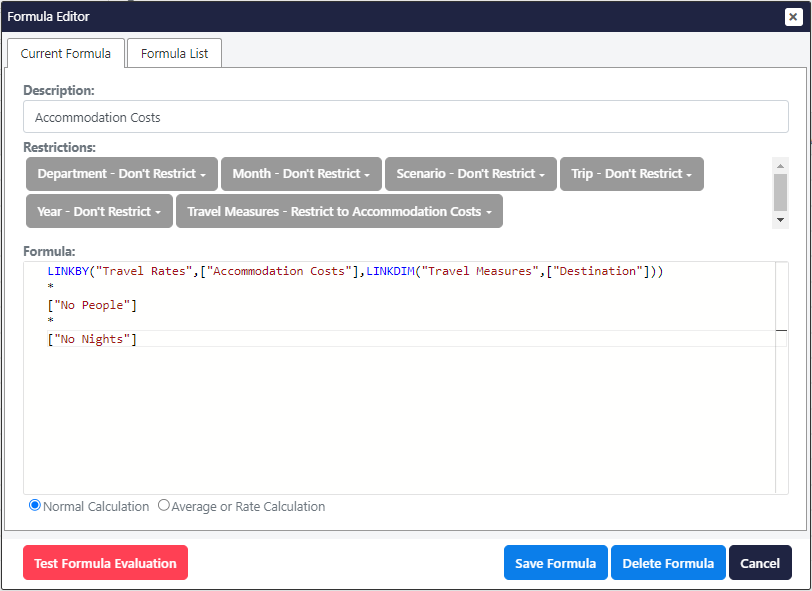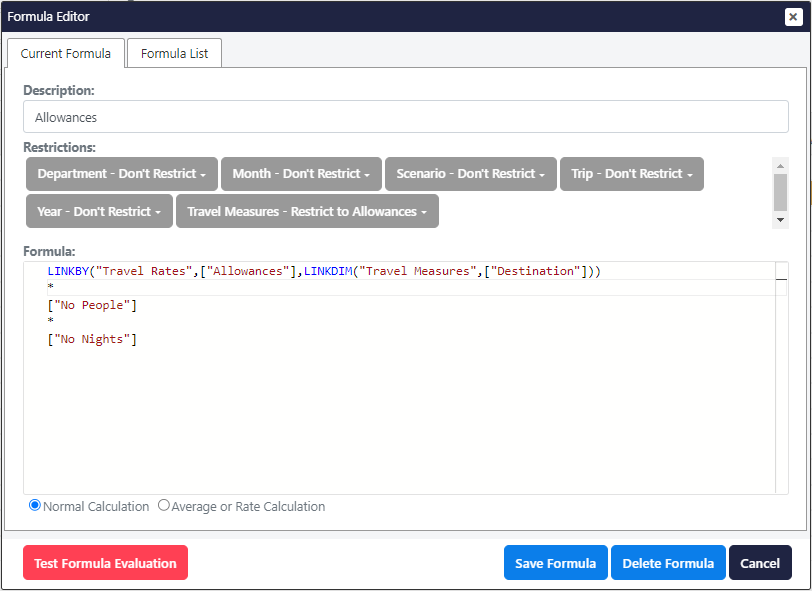To complete the Travel model, it will need to calculate Flight Costs, Accommodation Costs and Allowances while allowing Transit Expenses to be entered manually.

To open the formula editor right-click on a Flight Costs white cell and click Edit Formula.Within the Formula Editor, leave the restrictions as they are but copy the formula from below and paste this into the Formula field.

`LINKBY("Travel Rates",["Flight Costs"],LINKDIM("Travel Measures",["Destination"]))*["No People"]`

Click Save Formula to apply the formula.Right-click on an Accommodation Costs white cell and select Edit Formula. Within the Formula Editor, leave the restrictions as they are but copy the formula from below and paste this into the Formula field.

`LINKBY("Travel Rates",["Accommodation Costs"],LINKDIM("Travel Measures",["Destination"]))*["No People"]*["No Nights"]`

Click Save Formula to apply the formula.Right-click on a Allowances white cell and select Edit Formula. Within the Formula Editor, leave the restrictions as they are but copy the formula from below and paste this into the Formula field.

`LINKBY("Travel Rates",["Allowances"],LINKDIM("Travel Measures",["Destination"]))*["No People"]*["No Nights"]`

Click Save Formula to apply the formula.All the calculations should now be applied and you can change the Destination or other Measures values to test the new calculations.

Last updated: